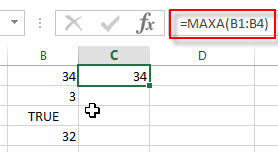# Excel MAXA Function

This post will guide you how to use Excel MAXA function with syntax and examples in Microsoft excel.

Description

The Excel MAXA function returns the largest numeric value from a range of values. And the MAXA function can be used to compare a range of values that contain numbers, text representations of numbers, or logical values. The logical value TRUE will be treated as value 1, the logical value FALSE will be treated as value 0. It will ignore the empty cells.

The MAXA function is a build-in function in Microsoft Excel and it is categorized as a Statistical Function.

The MAXA function is available in Excel 2016, excel 2013, excel 2010, excel 2007, excel 2003, excel XP, excel 2000, Excel 2011 for Mac.

### Syntax

The syntax of the MAXA function is as below:

`= MAXA(value1,[value2,…valueN])`

Where the MAXA function arguments are:

• value1-This is a required argument. The first number argument that you want to find the largest value, it can be numbers, test representations of numbers or logical values(TRUE, FALSE)
• value1,…valuen – This is an optional argument.  There can be up to 255 values for which you want to find the largest value.

Note:

• The arguments can be numbers, arrays, defined names or references that contain numbers, text representations of numbers, or logical values.
• If the arguments is an array or reference that contain empty cells or text values, and those values will be ignored.
• You can provide up to 255 number arguments in excel 2007 or later. If you are using excel 2003, you can only provide up to 30 number arguments.
• If the argument do not contain any values, the MAXA function will return 0.

### Excel MAXA Function Examples

The below examples will show you how to use Excel MAXA Function to get the largest value form the numbers in a range.

Example 1:   to get the largest value from a range B1:B4, just using the following excel formula:

`=MAXA(B1:B4)`### Related Functions

• Excel MAX function
The Excel MAX function returns the largest numeric value from the numbers that you provided. Or returns the largest value in the array.= MAX(num1,[num2,…numn])…